NCERT Solutions: Playing with Numbers- 2

# NCERT Solutions: Playing with Numbers- 2 Notes | Study Mathematics (Maths) Class 6 - Class 6

## Document Description: NCERT Solutions: Playing with Numbers- 2 for Class 6 2022 is part of Mathematics (Maths) Class 6 preparation. The notes and questions for NCERT Solutions: Playing with Numbers- 2 have been prepared according to the Class 6 exam syllabus. Information about NCERT Solutions: Playing with Numbers- 2 covers topics like Exercise 3.4, Exercise 3.5 and NCERT Solutions: Playing with Numbers- 2 Example, for Class 6 2022 Exam. Find important definitions, questions, notes, meanings, examples, exercises and tests below for NCERT Solutions: Playing with Numbers- 2.

Introduction of NCERT Solutions: Playing with Numbers- 2 in English is available as part of our Mathematics (Maths) Class 6 for Class 6 & NCERT Solutions: Playing with Numbers- 2 in Hindi for Mathematics (Maths) Class 6 course. Download more important topics related with notes, lectures and mock test series for Class 6 Exam by signing up for free. Class 6: NCERT Solutions: Playing with Numbers- 2 Notes | Study Mathematics (Maths) Class 6 - Class 6
 1 Crore+ students have signed up on EduRev. Have you?

Exercise 3.4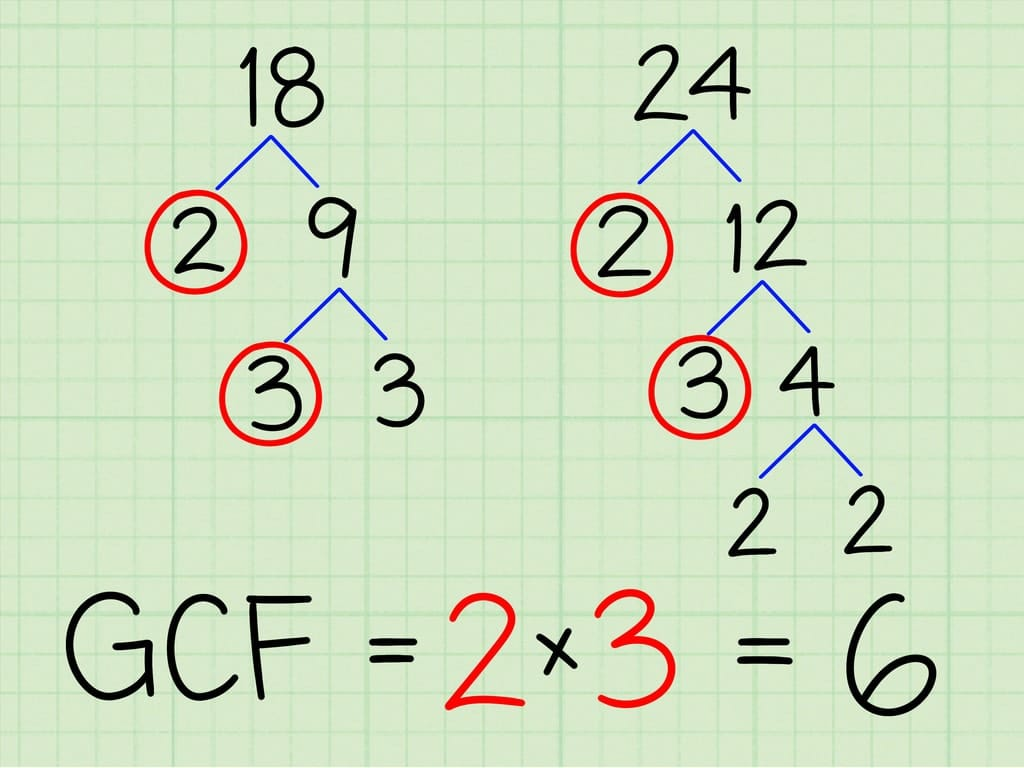Example for finding common factors

Ques 1: Find the common factors of:
(a) 20 and 28
(b) 15 and 25
(c) 35 and 50
(d) 56 and 120
Ans: (a) Factors of 20 = 1, 2, 4, 5, 10, 20
Factors of 28 = 1, 2, 4, 7, 14, 28
Common factors = 1, 2, 4
(b) Factors of 15 = 1, 3, 5, 15
Factors of 25 = 1, 5, 25
Common factors = 1, 5
(c) Factors of 35 = 1, 5, 7, 35
Factors of 50 = 1, 2, 5, 10, 25, 50
Common factors = 1, 5
(d) Factors of 56 = 1, 2, 4, 7, 8, 14, 28, 56
Factors of 120 = 1, 2, 3, 4, 5, 6, 8, 10, 12, 15, 20, 24, 30, 60, 120
Common factors = 1, 2, 4, 8

Ques 2: Find the common factors of:
(a) 4, 8 and 12
(b) 5, 15 and 25
Ans: (a) Factors of 4 = 1, 2, 4
Factors of 8 = 1, 2, 4, 8
Factors of 12 = 1, 2, 3, 4, 6, 12
Common factors of 4, 8 and 12 = 1, 2, 4
(b) Factors of 5 = 1, 5
Factors of 15 = 1, 3, 5, 15
Factors of 25 = 1, 5, 25
Common factors of 5, 15 and 25 = 1, 5

Ques 3: Find the first three common multiples of:
(a) 6 and 8
(b) 12 and 18
Ans: (a) Multiple of 6 = 6, 12, 18, 24, 30, 36, 42, 28, 54, 60, 72, …………
Multiple of 8 = 8, 16, 24, 32, 40, 48, 56, 64, 72, …………………….
Common multiples of 6 and 8 = 24, 48, 72
(b) Multiple of 12 = 12, 24, 36, 48, 60, 72, 84, 96, 108, 120, ………
Multiple of 18 = 18, 36, 54, 72, 90, 108, ………………………………
Common multiples of 12 and 18 = 36, 72, 108

Ques 4: Write all the numbers less than 100 which are common multiples of 3 and 4.
Ans: Multiple of 3 = 3, 6, 9, 12, 15, 18, 21, 24, 27, 30, 33, 36, 39, 42, 45, 48, 51, 54, 57, 60, 63, 66, 69, 72, 75, 78, 81, 84, 87, 90, 93, 96, 99
Multiple of 4 = 4, 8, 12, 16, 20, 24, 28, 32, 36, 40, 44, 48, 52, 56, 60, 64, 68, 72, 76, 80, 84, 88, 92, 96, 100
Common multiples of 3 and 4 = 12, 24, 36, 48, 60, 72, 84, 96

Ques 5: Which of the following numbers are co-prime:
(a) 18 and 35
(b) 15 and 37
(c) 30 and 415
(d) 17 and 68
(e) 216 and 215
(f) 81 and 16
Ans: (a) Factors of 18 = 1, 2, 3, 6, 9, 18
Factors of 35 = 1, 5, 7, 35
Common factor = 1
Since, both have only one common factor, i.e., 1, therefore, they are co-prime numbers.
(b) Factors of 15 = 1, 3, 5, 15
Factors of 37 = 1, 37
Common factor = 1
Since, both have only one common factor, i.e., 1, therefore, they are co-prime numbers.
(c) Factors of 30 = 1, 2, 3, 5, 6, 15, 30
Factors of 415 = 1, 5, …….., 83, 415
Common factor = 1, 5
Since, both have more than one common factor, therefore, they are not co-prime numbers.
(d) Factors of 17 = 1, 17
Factors of 68 = 1, 2, 4, 17, 34, 86
Common factor = 1, 17
Since, both have more than one common factor, therefore, they are not co-prime numbers.
(e) Factors of 216 = 1, 2, 3, 4, 6, 8, 36, 72, 108, 216
Factors of 215 = 1, 5, 43, 215
Common factor = 1
Since, both have only one common factor, i.e., 1, therefore, they are co-prime numbers.
(f) Factors of 81 = 1, 3, 9, 27, 81
Factors of 16 = 1, 2, 4, 8, 16
Common factor = 1
Since, both have only one common factor, i.e., 1, therefore, they are co-prime numbers.

Ques 6: A number is divisible by both 5 and 12. By which other number will that number be always divisible?
Ans: 5 x 12 = 60. The number must be divisible by 60.

Ques 7: A number is divisible by 12. By what other numbers will that number be divisible?
Ans: Factors of 12 are 1, 2, 3, 4, 6 and 12.
Therefore, the number also be divisible by 1, 2, 3 4 and 6.
Exercise 3.5
Ques 1: Which of the following statements are true:
(a) If a number is divisible by 3, it must be divisible by 9.
(b) If a number is divisible by 9, it must be divisible by 3.
(c) If a number is divisible by 18, it must be divisible by both 3 and 6.
(d) If a number is divisible by 9 and 10 both, then it must be divisible by 90.
(e) If two numbers are co-primes, at least one of them must be prime.
(f) All numbers which are divisible by 4 must also by divisible by 8.
(g) All numbers which are divisible by 8 must also by divisible by 4.
(h) If a number is exactly divides two numbers separately, it must exactly divide their sum.
(i) If a number is exactly divides the sum of two numbers, it must exactly divide the two numbers separately.
Ans: Statements (b), (c), (d), (g) and (h) are true.

Ques 2: Here are two different factor trees for 60. Write the missing numbers.
(a)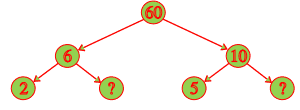(b)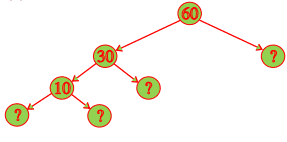(a)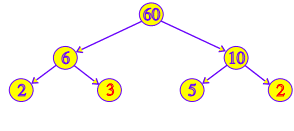(b)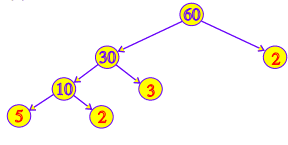Ques 3: Which factors are not included in the prime factorization of a composite number?
Ans: 1 is the factor which is not included in the prime factorization of a composite number.

Ques 4: Write the greatest 4-digit number and express it in terms of its prime factors.
Ans: The greatest 4-digit number = 9999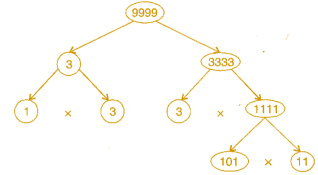The prime factors of 9999 are 3 × 3 × 11 × 101.

Ques 5: Write the smallest 5-digit number and express it in terms of its prime factors.
Ans: The smallest five digit number is 10000.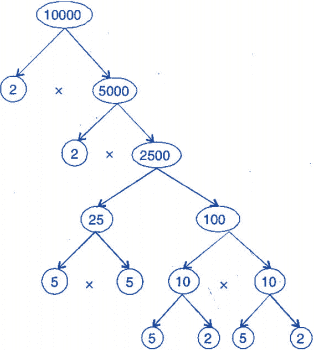The prime factors of 10000 are 2 × 2 × 2 × 2 × 5 × 5 × 5 × 5.

Ques 6: Find all the prime factors of 1729 and arrange them in ascending order. Now state the relation, if any, between, two consecutive prime numbers.
Ans: Prime factors of 1729 are 7 × 13 × 19.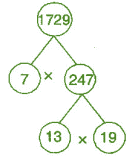The difference of two consecutive prime factors is 6.

Ques 7: The product of three consecutive numbers is always divisible by 6. Verify this statement with the help of some examples.
Ans: Among the three consecutive numbers, there must be one even number and one multiple of 3. Thus, the product must be multiple of 6.
Example: (i) 2 x 3 x 4 = 24
(ii) 4 x 5 x 6 = 120

Ques 8: The sum of two consecutive odd numbers is always divisible by 4. Verify this statement with the help of some examples.
Ans: 3 + 5 = 8 and 8 is divisible by 4.
5 + 7 = 12 and 12 is divisible by 4.
7 + 9 = 16 and 16 is divisible by 4.
9 + 11 = 20 and 20 is divisible by 4.

Ques 9: In which of the following expressions, prime factorization has been done:
(a) 24 = 2 x 3 x 4
(b) 56 = 7 x 2 x 2 x 2
(c) 70 = 2 x 5 x 7
(d) 54 = 2 x 3 x 9
Ans: In expressions (b) and (c), prime factorization has been done.

Ques 10: Determine if 25110 is divisible by 45.
[Hint: 5 and 9 are co-prime numbers. Test the divisibility of the number by 5 and 9.]
Ans: The prime factorization of 45 = 5 x 9
25110 is divisible by 5 as ‘0’ is at its unit place.
25110 is divisible by 9 as sum of digits is divisible by 9.
Therefore, the number must be divisible by 5 x 9 = 45

Ques 11: 18 is divisible by both 2 and 3. It is also divisible by 2 x 3 = 6. Similarly, a number is divisible by 4 and 6. Can we say that the number must be divisible by 4 x 6 = 24? If not, give an example to justify your answer.
Ans: No. Number 12 is divisible by both 6 and 4 but 12 is not divisible by 24.

Ques 12: I am the smallest number, having four different prime factors. Can you find me?
Ans: The smallest four prime numbers are 2, 3, 5 and 7.
Hence, the required number is 2 x 3 x 5 x 7 = 210

The document NCERT Solutions: Playing with Numbers- 2 Notes | Study Mathematics (Maths) Class 6 - Class 6 is a part of the Class 6 Course Mathematics (Maths) Class 6.
All you need of Class 6 at this link: Class 6

## Mathematics (Maths) Class 6

167 videos|275 docs|43 tests
 Use Code STAYHOME200 and get INR 200 additional OFF

## Mathematics (Maths) Class 6

167 videos|275 docs|43 tests

Track your progress, build streaks, highlight & save important lessons and more!

,

,

,

,

,

,

,

,

,

,

,

,

,

,

,

,

,

,

,

,

,

;